This chapter describes basic proration concepts.

## Calculating Prorated Cycle Fees

Oracle Communications Billing and Revenue Management (BRM) calculates the prorated fee for a specified period by multiplying the cycle fee defined in the applicable product by a scale based on the amount of time the product is valid during the cycle. The scale for a whole cycle (unit interval, or usually an accounting cycle) is equal to 1.

If a base product is valid during part of the cycle and one or more customized products is valid during another part of the cycle, BRM calculates the cycle fees for each product based on the proportion of time in the cycle that the products are valid. The total fee charged for the cycle is the sum of the individual charges calculated for each product that is valid during the cycle. See "Calculating Prorated Cycle Fees and Refunds for Customized Products".

BRM calculates the scale for a partial cycle by dividing the number of days in the partial cycle by the number of days in the unit interval within which it falls. The number of days in a unit interval will vary depending on its start and end dates.

If the period for which the prorated fee is being calculated is greater than one unit interval, the scale will be greater than 1. Likewise, if the period is less than one unit interval, the scale will be less than 1. For example, if the cycle fee is \$100, and the period being prorated is half a unit interval, the scale for that interval will be 0.5. So the prorated cycle fee is 0.5 * \$100 = \$50.

BRM does the following to calculate the prorated fee for each product that is valid during the cycle:

1. Calculates the unit intervals. See "Calculating the Unit Interval".

2. Calculates the scales for the part of the period to be prorated that falls into each unit interval.

3. Sums up the scales for all the unit intervals to get the scale for the entire period to be prorated.

4. Calculates the prorated amount by multiplying the scale for the entire period by the cycle fee amount.

### Calculating the Unit Interval

BRM takes into account what the use_number_of_days_in_month entry in the Connection Manager (CM) configuration (pin.conf) file is set to while calculating the unit interval. Also, the use of special days (29th, 30th, and 31st of a month) for billing is taken into account while calculating the unit interval.

Note:

If the timestamp_rounding entry in the CM pin.conf file is set to 0, the unit interval is calculated in seconds because the timestamp will not be rounded to midnight and the proration will begin from the time that is indicated by the timestamp. If timestamp_rounding is set to 1, the unit interval will be calculated in days because the timestamp will be rounded to midnight. For more information, see "About Time-Stamp Rounding."

#### Calculating Unit Interval When use_number_of_days_in_month Is Not Set or 0

If use_number_of_days_in_month is not set or is set to 0, the unit interval is calculated based on the billing time. Assuming the billing time to be March 22, starting from the billing time, BRM calculates the last unit interval by moving to the left (on the time axis) one month at a time until the beginning of the period to be prorated is covered. For example, while calculating the prorated fee for Mar 1–Mar 15, it takes the unit interval as 28 (the number of days between February 22 and March 22) because both March 1 and March 15 fall between February 22 and March 22. See "Example 1: Use_number_of_days_in_month Is Not Set or Set to 0".

#### Calculating Unit Interval When use_number_of_days_in_month Is Set to 1

If use_number_of_days_in_month is set to 1 and the period to be prorated falls within the same calendar month, the unit interval is the number of days in the whole calendar month in which the period to be prorated falls. For example, while calculating the prorated fee for Mar 1–Mar 15 (both dates fall in March), BRM calculates the unit interval as 31, because March has 31 days. See "Example 2: Use_number_of_days_in_month Is Set to 1".

Note:

If the period for which BRM is prorating the fees is less than a month but spans across multiple months (for example, Aug 19–Sep 15) or if the cycle fee is for multimonth, use_number_of_days_in_month is ignored.

#### Calculating Unit Interval When Billing Day of Month Is 29, 30, or 31

When the billing day of month (DOM) is set to 29, 30, or 31, the unit interval calculation is based on the option you set for this feature (the forward or back option set in the /config/business_params object. See "Setting the 31-Day Billing Feature".) If the option is set to forward and the month does not have the billing DOM, billing will run on the first day of the next month. If the option is set to back and the month does not have the billing DOM, billing will run on the last day of the previous month. This causes the start date of the unit interval to shift based on what option is set. See "Examples Using the 29th, 30th, and 31st for Billing Day of Month".

### Examples of Proration

Following are some examples of proration. In each of these examples, the unit interval is calculated differently. For additional examples, see "Calculating Prorated Cycle Fees and Refunds for Customized Products".

#### Example 1: Use_number_of_days_in_month Is Not Set or Set to 0

In this example, illustrated in Figure 4-1, the prorated cycle fee is calculated for the interval Feb 15–Apr 13 with the billing time as April 22. To calculate the prorated fee for this period, BRM does the following:

Calculates the Unit Intervals for the Period

Starting from the billing time (April 22), BRM calculates the last unit interval by moving to the left one month at a time (on the time axis). Therefore, the last unit interval will be Mar 22–Apr 22. Similarly, it will continue to calculate unit intervals until the start time of the first unit interval (January 22) will be equal to or before the start time of the given period to be prorated (February 15). As a result, it gets the following unit intervals:

Mar 22–Apr 22 (last unit interval)

Feb 22–Mar 22 (second unit interval)

Jan 22–Feb 22 (first unit interval)

Figure 4-1 Example 1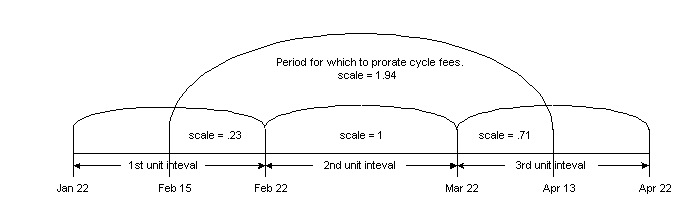Description of ''Figure 4-1 Example 1''

Calculates the Scale

To calculate the scale for the period Feb 15–Apr 13, BRM does the following:

1. Calculates the scale for the period Feb 15–Feb 22 with the unit interval as Jan 22–Feb 22.

Scale = 7/31 = 0.23

where 7 is the number of days between February 15 and February 22 and 31 is the number of days between January 22 and February 22.

2. Calculates the scale for the period Feb 22–Mar 22 with the unit interval as Feb 22–Mar 22. This scale is 28/28 = 1 because the period to be prorated is the unit interval.

3. Calculates the scale for the period Mar 22–Apr 13 with the unit interval as Mar 22–Apr 22.

Scale = 22/31 = 0.71

where 22 is the number of days between March 22 and April 13 and 31 is the number of days between March 22 and April 22.

4. Calculates the scale for the whole period Feb 15–Apr 13 as the sum of the scales from steps 1, 2, and 3: 0.23 + 1.0 + 0.71 = 1.94.

Calculates the Prorated Amount

If the cycle fee amount is \$100, BRM calculates the prorated amount for the period Feb 15–Apr 13 as follows:

1.94 * \$100 = \$194

#### Example 2: Use_number_of_days_in_month Is Set to 1

In this example, illustrated in Figure 4-2, the prorated cycle fee is calculated for the interval Feb 15–Apr 13 with the billing time as April 22. Because use_number_of_days_in_month is set to 1, BRM calculates the unit interval based on calendar month for the part of the proration time that falls in the same calendar month. To calculate the prorated fee for this period, it does the following:

Calculates the Unit Intervals for the Period

Starting from the billing time (April 22), BRM calculates the last unit interval by moving to the left one month at a time (on the time axis). Therefore, the unit intervals will be as follows:

Mar 22–Apr 22 (last unit interval)

Feb 22–Mar 22 (second unit interval)

Feb 1–Feb 28 (first unit interval)

Note:

This unit interval is the number of days in February because the entire period Feb 15–Feb 22 falls in February.

Figure 4-2 Example 2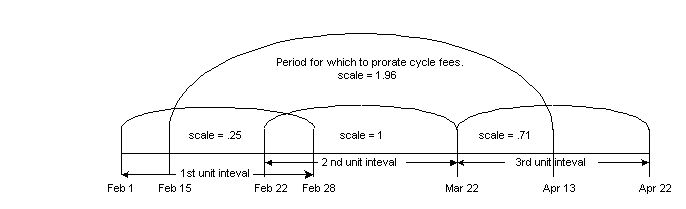Description of ''Figure 4-2 Example 2''

Calculates the Scale

To calculate the scale for the period Feb 15–Apr 13, BRM does the following:

1. Calculates the scale for the period Feb 15–Feb 22 with the unit interval as Feb 1–Feb 28.

Note:

If the part of the period to be prorated falls in the same calendar month, the unit interval is the number of days in the month. In this example, because the entire period Feb 15–Feb 22 falls in February, the unit interval is 28, the number of days in February.

Scale = 7/28 = 0.25

where 7 is the number of days between February 15 and February 22 and 28 is the number of days in February.

2. Calculates the scale for the period Feb 22–Mar 22 with the unit interval as Feb 22–Mar 22. This scale is 28/28 = 1 because the period to be prorated is the unit interval.

3. Calculates the scale for the period Mar 22–Apr 13 with the unit interval as Mar 22–Apr 22.

Scale = 22/31 = 0.71

where 22 is the number of days between March 22 and April 13 and 31 is the number of days between March 22 and April 22.

4. Calculates the scale for the whole period Feb 15–Apr 13 as the sum of the scales from steps 1, 2, and 3: 0.25 + 1.0 + 0.71 = 1.96.

Calculates the Prorated Amount

If the cycle fee amount is \$100, BRM calculates the prorated amount for the period Feb 15–Apr 13 as follows:

1.96 * \$100 = \$196

### Examples Using the 29th, 30th, and 31st for Billing Day of Month

The examples below show the use of special days (29th, 30th, 31st) as the billing DOM because of which the unit interval calculation will be based on the option you set for this feature (the forward or back option set in /config/business_params object). If the option is set to forward and the month does not have the billing DOM, billing is run on the first day of the next month. If the option is set to back and the month does not have the billing DOM, billing is run on the last day of the previous month. This causes the unit interval start date to shift based on the option set.

#### Example 3a: Use Forward Option with use_number_of_days_in_month Set to 0

This example, illustrated in Figure 4-3, assumes the billing option to be set to forward and use_number_of_days_in_month to be set to 0. To calculate the prorated cycle fee for the period Feb 15–Apr 13 with the billing day of month as April 30, BRM does the following:

Calculates the Unit Intervals for the Period

Starting from the billing time (April 30), BRM calculates the last unit interval by moving to the left (on the time axis) one month at a time. Therefore, the last unit interval will be Mar 30–Apr 30. Similarly, it continues to calculate unit intervals until the start time of the first unit interval (January 30) will be equal to or before the start time of the given period to be prorated (February 15). As a result, BRM gets the following unit intervals:

Mar 30–Apr 30 (last unit interval)

Mar 1–Mar 30 (second unit interval)

Note:

Because February does not have 30 days, and because the billing option is set to forward, BRM calculates this unit interval as beginning on the first day of the next month, which is March 1.

Jan 30–Mar 1 (first unit interval)

Note:

Because January does have 30 days, it calculates this unit interval as beginning on January 30.

Figure 4-3 Example 3a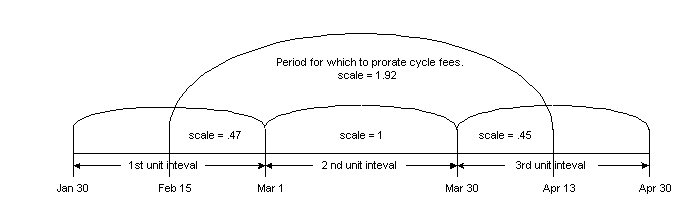Description of ''Figure 4-3 Example 3a''

Calculates the Scale

To calculate the scale for the period Feb 15–Apr 13, BRM does the following:

1. Calculates the scale for the period Feb 15–Mar 1 with the unit interval as Jan 30–Mar 1 as follows:

Scale = 14/30 = 0.47

where 14 is the number of days from February 15 and March 1 and 30 is the number of days from January 30 and March 1.

2. Calculates the scale for the period Mar 1–Mar 30 with the unit interval as Mar 1–Mar 30.

The scale for this period is 29/29 = 1 because number of days to be prorated is equal to number of days in the unit interval.

3. Calculates the scale for the period Mar 30–Apr 13 with the unit interval as Mar 30–Apr 30 as follows:

Scale = 14/31 = .45

where 14 is the number of days from March 30 and April 13 and 31 is the number of days from March 30 and April 30.

4. Adds the scales from the steps above to get the scale for the whole period:

Scale for the period Feb 15–Apr 13 = .47 + 1.0 + .45 = 1.92

Calculates the Prorated Cycle Fee Amount

If the cycle fee is \$100, the prorated cycle fee for the period Feb 15–Apr 13 will be:

1.92 * \$100 = \$192

#### Example 3b: Use Forward Option with use_number_of_days_in_month Set to 1

This example, illustrated in Figure 4-4, assumes the billing option to be set to forward and use_number_of_days_in_month to be set to 1. To calculate the prorated cycle fee for the period Feb 15–Apr 13 with the billing DOM as April 30, BRM does the following:

Calculates the Unit Intervals for the Period

Starting from the billing time (April 30), BRM calculates the last unit interval by moving to the left one month at a time (on the time axis). Therefore, the last unit interval will be Mar 30–Apr 30. Similarly, it continues to calculate unit intervals until the start time of the first unit interval (January 30) is equal to or before the start time of the given period to be prorated (February 15). As a result, BRM gets the following unit intervals:

Mar 30–Apr 30 (last unit interval)

Mar 1–Mar 31 (second unit interval)

Note:

Because February does not have 30 days, and because the billing option is set to forward, BRM calculates this unit interval as beginning on the first of the next month, which is March 1. Also, use_number_of_days_in_month is set to 1, it calculates this unit interval to be 31, the number of days in March.

Jan 30–Mar 1 (first unit interval)

Note:

Because January does have 30 days, this unit interval will begin on Jan 30.

Figure 4-4 Example 3b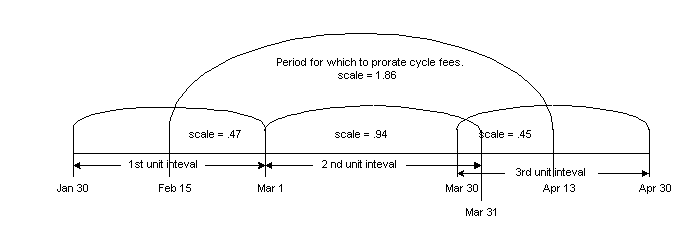Description of ''Figure 4-4 Example 3b''

Calculates the Scale

To calculate the scale for the period Feb 15–Apr 13, BRM does the following:

1. Calculates the scale for the period Feb 15–Mar 1 with the unit interval as Jan 30–Mar 1 as follows:

Scale = 14/30 = 0.47

where 14 is the number of days from February 15 and March 1 and 30 is the number of days between January 30 and March 1.

2. Calculates the scale for the period Mar 1–Mar 30 with the unit interval as Mar 1–Mar 31.

Note:

Because March 1 and March 30 fall in the same calendar month, the unit interval here will be the number of days in March, because use_number_of_days_in_month is set to 1.

Scale = 29/31 = .94

where 29 is the number of days between March 1 and March 30 and 31 is the number of days in March.

3. Calculates the scale for the period Mar 30–Apr 13 with the unit interval as Mar 30–Apr 30 as follows:

Scale = 14/31 = .45

where 14 is the number of days from March 30 and April 13 and 31 is the number of days between March 30 and April 30.

4. Adds the scales in the steps above to get the scale for the whole period:

Scale for the period Feb 15–Apr 13 = .47 + .94 + .45 = 1.86

Calculates the Prorated Cycle Fee Amount

If the cycle fee is \$100, the prorated cycle fee for the period Feb 15–Apr 13 will be:

1.86 * \$100 = \$186

#### Example 3c: Use Back Option with use_number_of_days_in_month Set to 1

This example, illustrated in Figure 4-5, assumes the billing option to be set to back and use_number_of_days_in_month to be set to 1. To calculate the prorated cycle fee for the period Feb 15–Apr 13 with the billing DOM as April 30, BRM does the following:

Calculates the Unit Intervals for the Period

Starting from the billing time (April 30), BRM calculates the last unit interval by moving to the left one month at a time (on the time axis). Therefore, the last unit interval will be Mar 30–Apr 30. Similarly, it continues to calculate unit intervals until the start time of the first unit interval will be equal to or before the start time of the given period (February 15) to be prorated. As a result, it gets the following unit intervals:

Mar 30–Apr 30 (last unit interval)

Feb 28–Mar 30 (second unit interval)

Note:

Because February does not have 30 days, and because the billing option is set to back, BRM calculates this unit interval as beginning on the last day of the previous month, which is February 28.

Feb 1–Feb 28 (first unit interval)

Note:

In this example, the first unit interval would have started on January 30, because January does have 30 days, but BRM ignores this and takes the number of days in February as the unit interval because use_number_of_days_in_month is set to 1.

Figure 4-5 Example 3c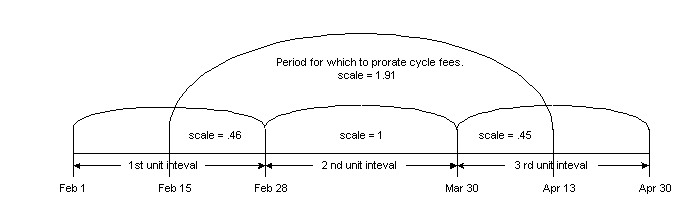Description of ''Figure 4-5 Example 3c''

Calculates the Scale

To calculate the scale for the period Feb 15–Apr 13, BRM does the following:

1. Calculates the scale for the period Feb 15–Feb 28 with the unit interval as Feb 1–Feb 28 as follows:

Scale = 13/28 = 0.46

where 13 is the number of days from February 15 and February 28 and 28 is the number of days in February.

Note:

Because February 15 and February 28 fall in the same calendar month, the unit interval here will be the number of days in February because use_number_of_days_in_month is set to 1.
2. Calculates the scale for the period Feb 28–Mar 30 with the unit interval as Feb 28–Mar 30.

The scale for this period is 30/30 = 1 because the number of days to be prorated is equal to number of days in the unit interval.

3. Calculates the scale for the period Mar 30–Apr 13 with the unit interval as Mar 30–Apr 30 as follows:

Scale = 14/31 = .45

where 14 is the number of days from March 30 and April 13 and 31 is the number of days between March 30 and April 30.

4. Adds the above three scales to get the scale for the whole period:

Scale for the period Feb 15–Apr 13 = .46 + 1.0 + .45 = 1.91

Calculates the Prorated Cycle Fee Amount

If the cycle fee is \$100, the prorated cycle fee for the period Feb 15–Apr 13 will be:

1.91 * \$100 = \$191

#### Example 3d: Use Back Option with use_number_of_days_in_month Set to 0

This example, illustrated in Figure 4-6, assumes the billing option to be set to back and use_number_of_days_in_month to be set to 0. To calculate the prorated fee for the period Feb 15–Apr 13 with the billing day of month as April 30, BRM does the following:

Calculates the Unit Intervals for the Period

Starting from the billing time (April 30), it calculates the last unit interval by moving to the left one month at a time (on the time axis). Therefore, the last unit interval will be Mar 30–Apr 30. Similarly, it continues to calculate unit intervals until the start time of the first unit interval will be equal to or before the start time of the given period to be prorated (February 15). As a result, BRM gets the following unit intervals:

Mar 30–Apr 30 (last unit interval)

Feb 28–Mar 30 (second unit interval)

Note:

Because February does not have 30 days, and because the billing option is set to back, BRM calculates this unit interval as beginning on the last day of the previous month, which is February 28.

Jan 30–Feb 28 (first unit interval)

Note:

Because January does have 30 days, BRM calculates this unit interval as beginning on January 30.

Figure 4-6 Example 3d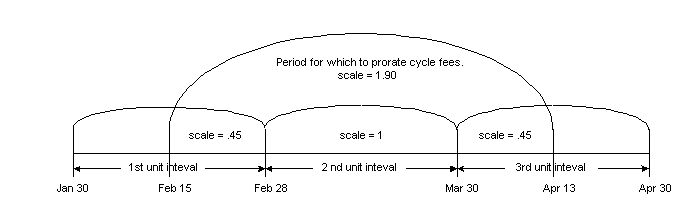Description of ''Figure 4-6 Example 3d''

Calculates the Scale

To calculate the scale for the period Feb 15–Apr 13, BRM does the following:

1. Calculates the scale for the period Feb 15–Feb 28 with the unit interval Jan 30–Feb 28:

Scale = 13/29 = 0.45

where 13 is the number of days from February 15 and February 28 and 29 is the number of days from January 30 and February 28.

2. Calculates the scale for the period Feb 28–Mar 30 with the unit interval as Feb 28–Mar 30.

The scale for this period is 30/30 = 1 because the number of days to be prorated is equal to number of days in the unit interval.

3. Calculates the scale for the period Mar 30–Apr 13 with the unit interval as Mar 30–Apr 30.

Scale = 14/31 = .45

where 14 is the number of days from March 30 and April 13 and 31 is the number of days from March 30 and April 30.

4. Adds the scales for the above steps to get the scale for the whole period:

Scale for the period Feb 15–Apr 13 = .45 + 1.0 + .45 = 1.90

Calculates the Prorated Cycle Fee Amount

If the cycle fee is \$100, the prorated cycle fee for the period Feb 15–Apr 13 will be:

1.90 * \$100 = \$190

## Enabling BRM to Apply Proration Rules

By default, BRM does not apply proration rules to product purchases or cancellations that align with the start of the product cycle. This allows you to apply full cycle fees or refunds for such purchases or cancellations irrespective of the proration rules set. For example, if start date of the product cycle is July 14 and the product is purchased on the same day, proration rules are not applied and full cycle fees are applied for that product. However, if the product is purchased on July 20, proration rules are applied and the cycle fee is prorated.

However, you can configure BRM to apply proration rules for all product purchases or cancellations irrespective of whether they align with the start of the product cycle or not. For example, you can prevent a refund for a fee even if the full cycle is being refunded by applying proration rules. You can configure this by enabling the ApplyProrationRules business parameter in the subscription instance of the /config/business_params object.

To enable BRM to apply proration rules:

1. Go to the BRM_home/sys/data/config directory, where BRM_home is the directory in which you installed the BRM software.

2. Run the following command, which creates an editable XML file from the subscription instance of the /config/business_params object:

```pin_bus_params -r BusParamsSubscription bus_params_subscription.xml
```

This command creates the XML file named bus_params_subscription.xml.out in your working directory. To place this file in a different directory, specify the path as part of the file name.

3. Open the bus_params_subscription.xml.out file in a text editor.

4. Search the file for the following line:

```<ApplyProrationRules>disabled</ApplyProrationRules>
```

By default, the ApplyProrationRules parameter is disabled.

5. Change disabled to enabled.

6. Save this file as bus_params_subscription.xml.

7. Go to the BRM_home/sys/data/config directory, which includes support files used by the pin_bus_params utility.

8. Run the following command, which loads this change into the /config/business_params object:

```pin_bus_params PathToWorkingDirectory/bus_params_subscription.xml
```

where PathToWorkingDirectory is the directory in which bus_params_subscription.xml resides.

9. Read the object with the testnap utility or Object Browser to verify that all fields are correct.

For more information on reading objects by using Object Browser, see BRM Managing Customers. For instructions on using the testnap utility, see BRM Developer's Guide.

## Proration for Special Cases

BRM includes proration settings that address customers with cycle arrears fees who purchase, inactivate, and reactivate products within the first cycle.

You choose the rating behavior when such events occur by setting the cycle_arrear_proration parameter in the CM configuration (pin.conf) file:

```- fm_rate cycle_arrear_proration = 0|1
```

Important:

You must restart the CM after you change this parameter. See "Starting and Stopping the BRM System" in BRM System Administrator's Guide.

When you set up proration in a product's rate plan, you choose a proration setting for when the product is purchased and for when the product is canceled:

• If you want the proration setting for purchase time used to rate periods in which a customer both purchases and inactivates a product, set the cycle_arrear_proration parameter to 0.

• If you want the cancellation proration setting used to rate periods in which a customer cancels a product, set the cycle_arrear_proration parameter to 1.

### Special Cases

Some special proration cases may result in unexpected billing results:

• A customer purchases a product with a cycle arrears fee, inactivates the product, and then reactivates the product all in the same accounting cycle. The product is set to prorate on purchase and charge full on cancellation, and the cycle_arrear_proration entry in the CM pin.conf is set to 1.

In this case, instead of charging a prorated amount based on the total time the customers product was active during the first cycle, the full amount for the cycle is charged.

• A customer purchases a product with a cycle arrears fee, inactivates the product, and then reactivates the product all in the same accounting cycle. The product is set to no charge on purchase and prorate on cancellation, and the cycle_arrear_proration entry in the CM pin.conf is set to 1.

In this case, instead of being charged nothing for the first accounting cycle, the customer is charged a prorated amount based on the time the product was active, except for the time of the last active period in the cycle.

For example, if the customer purchases a product, inactivates it after 5 days, reactivates it later for another period of 5 days before inactivating it, and then reactivates it later for a total of 8 days before the cycle ends, you charge the customer for (5 + 5), or 10 days of use.

• A customer purchases a product with a cycle arrears fee, inactivates the product, and then reactivates the product all in the same accounting cycle. The product is set to charge full on purchase and prorate on cancellation, and the cycle_arrear_proration entry in the CM pin.conf is set to 1.

In this case, instead of being charged for the whole accounting cycle, the customer is charged a prorated amount based on the total time during the cycle that the product was active.

• With a cycle arrears fee, the customer inactivates and reactivates a product in a cycle other than the cycle in which the product was purchased. The product has proration settings of charge full, no charge, or prorate on purchase, and charge full or no charge on product cancellation.

In this case, the customer is charged a prorated amount equal to the time the product was active during the accounting cycle from the last reactivation to the end of the cycle. There is no charge for all other active time periods during the accounting cycle.

• With cycle forward billing, the customer purchases a product, inactivates the product, and reactivates the product in the same period. The product has proration settings of charge full on purchase and prorate on cancellation.

In this case, instead of being charged for the whole accounting cycle, the customer is charged for the whole cycle less the period of time when the product was inactivated.

Note:

• You can set your policy settings so that customers are not charged for short usage periods. This establishes the possibility of long cycles over 30 days long for which a customer is not billed.

• For the proration settings Charge for the full cycle on purchase and Don't charge this cycle on cancellation, a cycle is always ”1” or less, even if a customer purchases and cancels within a long cycle.

• The proration settings Charge for the full cycle, Don't charge this cycle, and Charge based on usage for the inactivation/cancellation of a product when you are using cycle forward billing refers to refunding. Charge for the full cycle means you refund nothing on inactivation/cancellation, Don't charge this cycle means you refund all money charged for the cycle, and Charge based on usage means you refund the unused portion of the monthly usage fee.

There are several techniques you can use to address any special cases that may occur when customers inactivate and reactivate an account in the same accounting cycle:

• Inform your customers of the discrepancies.

• Do not allow your customer service representatives (CSRs) to inactivate and reactivate accounts frequently, especially during the first accounting cycle in which a product is purchased.

• Because most special cases occur when you use cycle arrears fees, consider using cycle forward fees instead.

## Calculating Prorated Cycle Fees and Refunds for Customized Products

When a customized product is valid during all or part of a cycle, BRM uses it to calculate prorated cycle fees and refunds. See "About Customized Product Validity" in BRM Managing Customers.

• When a customized product is valid during the entire cycle, proration occurs just as with any other product.

• When a base product is valid during part of the cycle and one or more customized products is valid during part of the cycle, BRM calculates the fee or refund based on the proportion of time that each product is valid during the cycle or refund period.

### Example 4: Applying a Cycle Forward Fee with a Customized Product

In this example, illustrated in Figure 4-7, Product A has a monthly cycle forward fee of \$12. Its cycle fees are effective from January 1 to December 31. A CSR customizes the product to reduce the cycle forward fee by 50% for the period Apr 10–Apr 20.

Figure 4-7 Example 4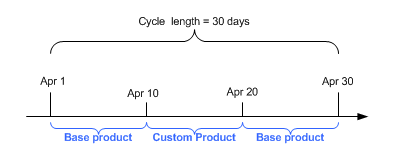Description of ''Figure 4-7 Example 4''

BRM calculates the total cycle fee for the month of April by calculating the fees separately for the portions that are covered by the base product (Product A's standard fee) and the customized product (the 50% reduction).

For each product, BRM calculates the scale by comparing the number of days in the cycle to the number of days in the cycle that the product is valid. For April, the number of days in the cycle is 30.

• The base product is valid from Apr 1–Apr 10 and Apr 21–Apr 30, a total of 20 days. Its scale is therefore 20/30. The base product's cycle fee is \$12, so its share of the total cycle fee is 20/30 * \$12 = \$8.

• The customized product is valid from Apr 11–Apr 20, a total of 10 days. Its scale is 10/30. The customized product's cycle fee is \$10, so its share of the cycle fee is 10/30 * \$6 = \$2.

The total cycle fee is the sum of the two individually calculated fees, or \$10.

### Example 5: Refunding a Prorated Cycle Forward Fee with a Customized Product

In this example, illustrated in Figure 4-8, the customer cancels the account that owns Product A and its customization on April 15. Cycle fee proration is set to Calculate the charge based on the amount used, so BRM must calculate a prorated refund that includes both the base product and the customized product.

Figure 4-8 Example 5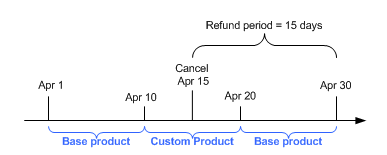Description of ''Figure 4-8 Example 5''

For this calculation, BRM begins with the scale that was originally applied to the cycle fee for each product. For the base product, this is 20/30; and for the customized product, 10/30.

BRM then calculates the proportion of the days that were used to calculate the scale that fall within the refund period. In this case, the refund period is Apr 15–Apr 30.

• For the base product, 10 days (Apr 21–Apr 30) of the period that was used to calculate the original scale fall within the refund period. So the proportion is 10/20.

• For the customized product, 5 days (Apr 11–Apr 15) of the period that was used to calculate the original scale fall within the refund period. So the proportion is 5/10.

To calculate the refund, BRM multiplies the rate by product of the original scale and the proportion of the scale that falls within the refund period.

• For the base product, the refund is \$12 * 20/30 * 10/30 = \$4.

• For the customized product, the refund is \$6 * 10/30 * 5/10 = \$1.

The total refund is \$4 + \$1 = \$5.

To work in parallel with older legacy billing systems, you can use 30-day-based proration.

In older legacy billing systems, it is common to use 30 days for calculating proration, irrespective of the actual number of days in the month or the billing cycle. By default, BRM calculates proration based on the number of days in the billing cycle. You use 30-day-based proration when you have BRM with an older billing system requiring 30-day-based proration.

Important:

Making 30-day-based proration work with a normal calendar year can cause unexpected behavior. See "Special Cases".

Note:

30-day-based proration cannot be used with multimonth billing cycles.

### Examples of 30-Day-Based Proration

Based on your proration setting, BRM calculates the prorated cycle amount differently, which may yield different results.

In the examples below, illustrated in Figure 4-9, Figure 4-10, Figure 4-11, and Figure 4-12, February has 28 days and billing occurs on the second day of every month. The monthly cycle forward fee is \$30 and timestamp_rounding is set to 1 days in the CM pin.conf file.

#### Example 6: Prorated Purchase Fee with 31-day Billing Cycle

Figure 4-9 Example 6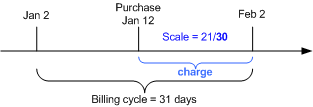Description of ''Figure 4-9 Example 6''

In this example, BRM calculates the prorated cycle fee as follows:

Using 30-Day proration

1. Calculates the scale using 30 days as the base:

scale = 21/30 = .70

where 21 is the number of days from midnight January 12 to midnight February 2.

2. Calculates the prorated cycle fee:

cycle fee amount * scale = \$30.00 * .70 = \$21.00

Using 31 Days in the Billing Cycle

1. Calculates the scale using 31 days as the base:

scale = 21/31 = .68

where 21 is the number of days from midnight January 12 to midnight February 2.

2. Calculates the prorated cycle fee:

cycle fee amount * scale = \$30.00 * .68 = \$20.32

#### Example 7: Prorated Cancel Fee with 31-day Billing Cycle

Figure 4-10 Example 7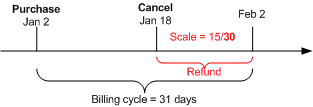Description of ''Figure 4-10 Example 7''

In this example, BRM calculates the cycle fee refund as follows:

Using 30-Day Proration

1. Calculates the scale using 30 days as the base:

scale = 15/30 = .50

where 15 is the number of days from midnight January 18 to midnight February 2.

2. Calculates the refund amount:

cycle fee amount * scale = \$30.00 * .50 = \$15.00

Using 31 Days in the Billing Cycle

1. Calculates the scale using 31 days as the base:

scale = 15/31 = .48

where 15 is the number of days from midnight January 18 to midnight February 2.

2. Calculates the refund amount:

cycle fee amount * scale = \$30.00 * .48 = \$14.52

#### Example 8: Prorated Purchase Fee with 28-Day Billing Cycle

Figure 4-11 Example 8Description of ''Figure 4-11 Example 8''

In this example, BRM calculates the prorated cycle fee as follows:

Using 30-Day Proration

1. Calculates the scale using 30 days as the base:

scale = 15/30 = .50

where 15 is the number of days from midnight February 15 to midnight March 2.

2. Calculates the prorated cycle fee:

cycle fee amount * scale = \$30.00 * .50 = \$15.00

Using 28 Days in the Billing Cycle

1. Calculates the scale using 28 days as the base:

scale = 15/28 = .54

where 15 is the number of days from midnight February 15 to midnight March 2.

2. Calculates the prorated cycle fee:

cycle fee amount * scale = \$30.00 * .54 = \$16.07

#### Example 9: Prorated Cancel Fee with 28-Day Billing Cycle

Figure 4-12 Example 9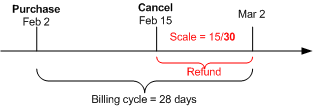Description of ''Figure 4-12 Example 9''

In this example, BRM calculates the cycle fee refund as follows:

Using 30-Day Proration

1. Calculates the scale using 30 days as the base:

scale = 15/30 = .50

where 15 is the number of days from midnight January 18 to midnight February 2.

2. Calculates the refund amount:

cycle fee amount * scale = \$30.00 * .50 = \$15.00

Using 28 Days in the Billing Cycle

1. Calculates the scale using 28 days as the base:

scale = 15/28 = .54

where 15 is the number of days from midnight January 18 to midnight February 2.

2. Calculates the refund amount:

cycle fee amount * scale = \$30.00 * .54 = \$16.07

### Special Cases

When using 30-day proration, there can be unexpected results, as shown in the following examples:

#### Example 10: Full Purchase Fee Charged When Service Is Provided for 1 Day Less

In this example, illustrated in Figure 4-13, the billing cycle is 31 days. The customer purchases the product on January 3, so the scale is 1. The customer pays the full \$30 cycle fee even though the service was available for one day less.

Figure 4-13 Example 10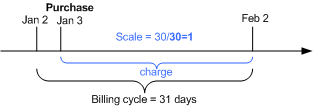Description of ''Figure 4-13 Example 10''

The prorated cycle fee:

cycle fee * scale = \$30.00 * 1 = \$30.

#### Example 11: Full Cancel Fee Refunded When Service Has Been Used for 1 Day

In this example, illustrated in Figure 4-14, the billing cycle is 31 days and the monthly service fee is \$30. The customer purchases the product on January 1 and cancels the product on January 2. The scale is 1. The customer gets a full \$30 refund even though customer owned the product for one day.

Figure 4-14 Example 11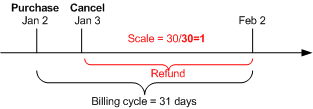Description of ''Figure 4-14 Example 11''

The refund amount is:

cycle fee * scale = \$30.00 * 1 = \$30.

Important:

To avoid such situations, use the number of days in the billing cycle or number of days in the month proration settings, not the 30-day proration setting.

### Enabling 30-Day-Based Proration

To enable 30-day-based proration:

1. Open the CM configuration file (BRM_Home/sys/cm/pin.conf). BRM_Home is the directory where you installed BRM components.

2. Edit the following entry:

```-fm_bill enable_30_day_proration 0
```

where:

• 0 bases proration on the number of days in the billing cycle or number of days in the month. This is the default.

• 1 bases proration on a 30-day month.

3. Save and close the file.

## Using Two Events to Prorate Charges for Products Whose Validity Ends in First Cycle

When prorating a cycle fee for a product whose cycle validity period ends during the same billing cycle in which the product was purchased, BRM can generate one or two events:

• One event: By default, BRM generates a single event to charge for the used portion of the cycle.

• Two events: BRM generates an event to charge for the entire cycle and then generates an event to refund the charge for the unused portion of the cycle (product cycle validity end date through billing cycle end date).

To use two events, enable the CreateTwoEventsInFirstCycle business parameter in the subscription instance of the /config/business_params object with the pin_bus_param utility.

For example, by default, if the product is purchased on January 1 and the product's cycle validity period ends on January 16, only one event is generated for a prorated cycle fee for the number of days from midnight January 1 to midnight January 16. When the CreateTwoEventsInFirstCycle business parameter is enabled, the first event is generated for a cycle fee for the number of days from midnight January 1 to midnight February 1 and a second event is generated for a refund for the number of days from midnight January 16 to midnight February 1.

To use two events to prorate a cycle fee for a product whose cycle validity period ends in the first cycle:

1. Go to the BRM_Home/sys/data/config directory, where BRM_Home is the directory in which you installed BRM.

2. Run the following command, which creates an editable XML file from the subscription instance of the /config/business_params object:

```pin_bus_params -r BusParamsSubscription bus_params_subscription.xml
```

This command creates the XML file named bus_params_subscription.xml.out in your working directory. To place this file in a different directory, specify the path as part of the file name.

3. Open the bus_params_subscription.xml.out file in a text editor.

4. Search the file for the following line:

```<CreateTwoEventsInFirstCycle>disabled</CreateTwoEventsInFirstCycle>
```

By default, the CreateTwoEventsInFirstCycle parameter is disabled.

5. Change disabled to enabled.

6. Save this file as bus_params_subscription.xml.

7. Go to the BRM_Home/sys/data/config directory, which includes support files used by the pin_bus_params utility.

8. Run the following command, which loads this change into the /config/business_params object:

```pin_bus_params PathToWorkingDirectory/bus_params_subscription.xml
```

where PathToWorkingDirectory is the directory in which bus_params_subscription.xml resides.

Caution:

BRM uses the XML in this file to overwrite the existing Subscription instance of the /config/business_params object. If you delete or modify any other parameters in this file, your changes affect the associated aspects of the BRM configuration.

Note:

To run this command from a different directory, see "pin_bus_params" in BRM Developer's Guide.
9. Read the object with the testnap utility or Object Browser to verify that all fields are correct.

10. Stop and restart the CM. For more information, see the description of starting and stopping the BRM System in BRM System Administrator's Guide.

## Prorating Cycle Fees after a Discount Purchase or Cancellation

When off-cycle discounts are purchased or canceled, and the discount's usage map is configured to support any of the product's cycle forward event types, the cycle fees are discounted for the duration of the cycle for which the discounts are valid.

### Examples of Cycle Fee Proration

These examples show how cycle fee proration works.

#### Example 12: Cycle Fee Is Refunded after a Discount Purchase

In this example, illustrated in Figure 4-15, a \$30 monthly cycle fee is charged on January 1. An off-cycle discount is purchased on January 15 that discounts 10% of the monthly fee. The 10% discount is applied to the prorated monthly charge of \$15 from Jan 15–Feb 1. A refund amount of \$1.50 is credited to the account or service balance.

Figure 4-15 Example 12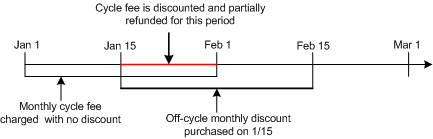Description of ''Figure 4-15 Example 12''

#### Example 13: Free Minutes Are Prorated after a Discount Cancellation

In this example, illustrated in Figure 4-16, a cycle forward monthly event grants 100 free minutes. The discount is canceled on January 15. The account made 200 minutes of calls between January 1 and January 15 at \$0.10 per minute. The 100 free minutes are prorated between January 1 and January 15. The account is granted 50 free minutes and is charged \$0.10 per minute for the remaining 150 minutes.

Figure 4-16 Example 13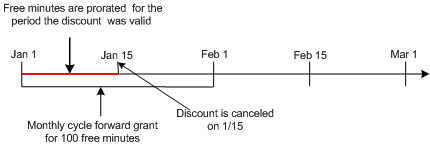Description of ''Figure 4-16 Example 13''

#### Example 14: Canceled Discount Proration Is Not Taken into Account When Product Is Canceled

If you cancel a discount for a cycle fee, and then you cancel the product that owns that cycle fee, the prorated cycle fee is refunded without considering any discount that was applied. Therefore, the refunded amount might be more than the charged amount. However, this can be corrected by rerating the cycle event.

In this example, an account owns a product with a cycle fee of \$60, cancellation proration settings set to Do not charge for the cycle, and a discount of 10% on the cycle fee. At the beginning of the cycle, \$54 is charged (\$60 cycle fee minus \$6 discount). In the middle of the cycle, if the discount is canceled, and \$3 is charged back. Later, if the product is canceled, and \$60 is refunded (because the cancellation proration setting is Do not charge for the cycle). Therefore, the net charge for the cycle is a credit of \$3. If you rerate the events for the cycle, the expected charge of \$60 will be applicable.

## Prorating Cycle Fees When a Discount's Cycle Start or End Date Is Changed

When a discount's cycle start or end dates are changed, cycle fees may be discounted and refunded or charged depending on the new cycle start and end dates.

### Examples of Cycle Fee Proration

These examples show how cycle fee proration works.

#### Example 15: Cycle Fee Is Refunded When a Discount's Cycle Start Date Is Changed

In this example, illustrated in Figure 4-17, a monthly cycle fee is charged on January 1. The monthly fee is discounted from Jan 20–Feb 1, and the discounted amount is credited to the account balance. When the discount cycle start date is changed to January 10, the monthly fee is discounted from Jan 10–Jan 20, and the discounted amount is credited to the account balance.

Figure 4-17 Example 15Description of ''Figure 4-17 Example 15''

#### Example 16: Cycle Fee Is Charged When a Discount's Cycle Start Date Is Changed

In this example, illustrated in Figure 4-18, a monthly cycle fee is charged on January 1. The monthly fee is discounted from Jan 10–Feb 1, and the discounted amount is credited to the account balance. When the discount cycle start date is changed to January 20, the discounted cycle fee amount is charged from Jan 10–Jan 20 because the discount is no longer valid during this period.

Figure 4-18 Example 16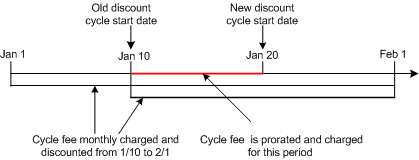Description of ''Figure 4-18 Example 16''

#### Example 17: Cycle Fee Is Refunded When Discount's Cycle End Date Is Changed

In this example, illustrated in Figure 4-19, a monthly cycle fee is charged on January 1. The monthly fee is discounted from Jan 1–Jan 10. When the discount cycle end date is changed to January 20, the cycle fee is discounted from Jan 10–Jan 20, and the discounted amount is credited to the account balance.

Figure 4-19 Example 17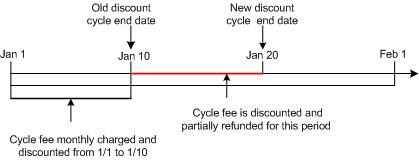Description of ''Figure 4-19 Example 17''

#### Example 18: Cycle Fee Is Charged When Discount's Cycle End Date Is Changed

In this example, illustrated in Figure 4-20, a monthly cycle fee is charged on January 1. The monthly fee is discounted from Jan 1–Jan 20. When the discount cycle end date is changed to January 10, the discounted cycle fee amount is charged from Jan 10–Jan 20.

Figure 4-20 Example 18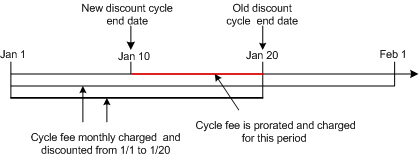Description of ''Figure 4-20 Example 18''